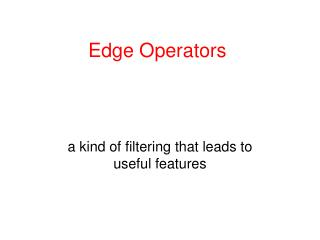DownloadDownload PresentationEdge Operators

# Edge Operators

Télécharger la présentation## Edge Operators

- - - - - - - - - - - - - - - - - - - - - - - - - - - E N D - - - - - - - - - - - - - - - - - - - - - - - - - - -
##### Presentation Transcript

1. Edge Operators a kind of filtering that leads to useful features

2. Lines and ArcsSegmentation In some image sets, lines, curves, and circular arcs are more useful than regions or helpful in addition to regions. • Lines and arcs are often used in • object recognition • stereo matching • document analysis

3. Edge Detection Basic idea: look for a neighborhood with strong signs of change. • Problems: • neighborhood size • how to detect change 81 82 26 24 82 33 25 25 81 82 26 24

4. Differential Operators • Differential operators • attempt to approximate the gradient at a pixel via masks • threshold the gradient to select the edge pixels

5. Example: Sobel Operator -1 0 1 1 2 1 Sx = -2 0 2 Sy = 0 0 0 -1 0 1 -1 -2 -1 • On a pixel of the image I • let gx be the response to Sx • let gy be the response to Sy Then the gradient is I = [gx gy] T And g = (gx2 + gy2 )1/2 is the gradient magnitude.  = atan2(gy,gx) is the gradient direction.

6. Sobel Operator on the Blocks Image original image gradient thresholded magnitude gradient magnitude

7. Common Masks for Computing Gradient • Sobel: • Prewitt: • Roberts -1 0 1 -2 0 2 -1 0 1 • 2 1 • 0 0 0 • -1 -2 -1 -1 0 1 -1 0 1 -1 0 1 • 1 1 • 0 0 0 • -1 -1 -1 0 1 -1 0 • 0 • 0 -1 Sx Sy

8. Zero Crossing Operators Motivation: The zero crossings of the second derivative of the image function are more precise than the peaks of the first derivative. step edge smoothed 1st derivative zero crossing 2nd derivative

9. How do we estimate the Second Derivative? • Laplacian Filter:  f =  f / x +  f / y 2 2 2 2 2 0 1 0 1 -4 1 0 1 0 • Standard mask implementation • Derivation: In 1D, the first derivative • can be computed with mask [-1 0 1] • The 1D second derivative is [1 -2 1] • The Laplacian mask estimates the • 2D second derivative.

10. Properties of Derivative Masks • Coordinates of derivative masks have opposite signs in order to obtain a high response in regions of high contrast. • The sum of coordinates of derivative masks is zero, so that a zero response is obtained on constant regions. • First derivative masks produce high absolute values at points of high contrast. • Second derivative masks produce zero-crossings at points of high contrast.

11. Marr/Hildreth Operator • First smooth the image via a Gaussian convolution. • Apply a Laplacian filter (estimate 2nd derivative). • Find zero crossings of the Laplacian of the Gaussian. • This can be done at multiple resolutions.

12. Haralick Operator • Fit the gray-tone intensity surface to a piecewise • cubic polynomial approximation. • Use the approximation to find zero crossings of the • second directional derivative in the direction that • maximizes the first directional derivative. • The derivatives here are calculated from direct • mathematical expressions wrt the cubic polynomial.

13. Canny Edge Detector • Smooth the image with a Gaussian filter with spread. • Compute gradient magnitude and direction at each pixel of • the smoothed image. • Zero out any pixel response  the two neighboring pixels • on either side of it, along the direction of the gradient. • Track high-magnitude contours. • Keep only pixels along these contours, so weak little • segments go away.

14. Canny on Kidney

15. Canny Characteristics • The Canny operator gives single-pixel-wide images with good continuation between adjacent pixels • It is the most widely used edge operator today; no one has done better since it came out in the late 80s. Many implementations are available. • It is very sensitive to its parameters, which need to be adjusted for different application domains.